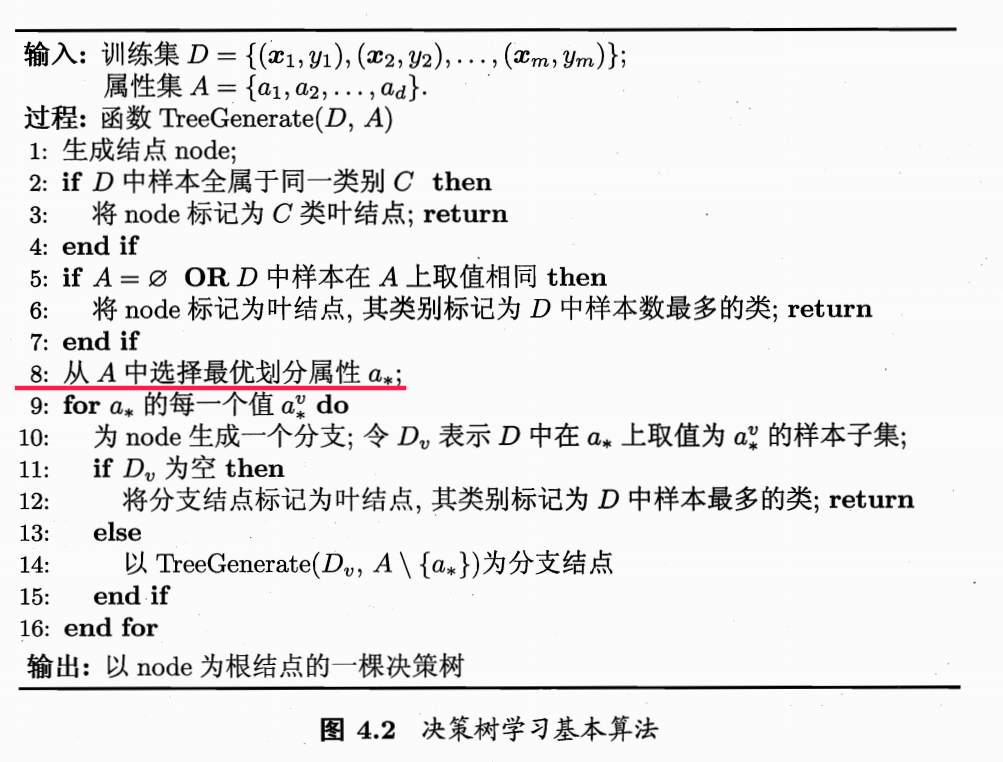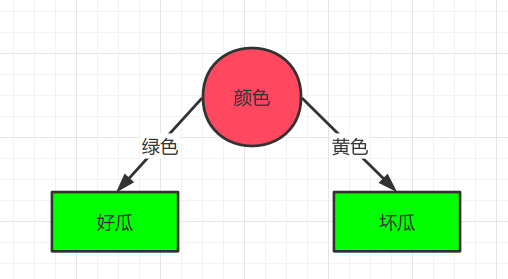# 动手机器学习->实现决策树

1. 划分选择
2. 决策树的生成

## 二、划分选择

$$Ent(D) = - ( \frac{1}{3} log_2\frac{1}{3} + \frac{2}{3} log_2\frac{2}{3} ) = 0.918$$

$$Ent(D) = - ( \frac{1}{2} log_2\frac{1}{2} + \frac{1}{2} log_2\frac{1}{2} ) = 1$$

 1 2 3 4 5 6 7  import numpy as np def entropy(y): ## y是事件的结果，比如抛硬币1次正，2次反.y就为[1,2,2] hist = np.bincount(y) ps = hist / np.sum(hist) return -np.sum([p * np.log2(p) for p in ps if p > 0]) 

## 三、决策树的生成1. 如果数据集中样本为同一类别或者没有特征可以继续划分，停止划分为叶结点赋值
2. 选择最优划分属性
3. 以最优属性将原数据集分为两个数据集，递归构建决策树

1 绿色
2 不响 绿色
3 黄色 不是

$$Ent(敲声) = - \frac{2}{3} (\frac{1}{2} log_2 \frac{1}{2} + \frac{1}{2} log_2 \frac{1}{2}) - \frac{1}{3} (\frac{1}{1} log_2 \frac{1}{1} + \frac{0}{1} log_2 \frac{0}{1}) = 0.666 + 0 = 0.666$$

Warning

$$Ent(颜色) = - \frac{2}{3} (\frac{2}{2} log_2 \frac{2}{2} + \frac{0}{2} log_2 \frac{0}{2}) - \frac{1}{3} (\frac{0}{1} log_2 \frac{0}{1} + \frac{1}{1} log_2 \frac{1}{1}) = 0 + 0 = 0$$